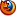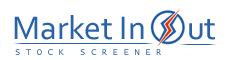Please enable JavaScript to view this page content properly Log In | Sign Up
 MACD MACD Histogram PPO PPO Histogram Parabolic SAR ADX Aroon Accumulation / Distribution William's Acc / Distr Choppiness Index Balance Step SuperTrend Vortex WaveTrend Force
 ADX Action -DI(7) is Below +DI(7)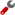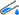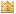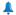-DI(14) is Below +DI(14)-DI(28) is Below +DI(28)-DI(7) is Above +DI(7)-DI(14) is Above +DI(14)-DI(28) is Above +DI(28)-DI(7) Crossed Below +DI(7)-DI(14) Crossed Below +DI(14)-DI(28) Crossed Below +DI(28)-DI(7) Crossed Above +DI(7)-DI(14) Crossed Above +DI(14)-DI(28) Crossed Above +DI(28)ADX(7) is Above 25ADX(14) is Above 25ADX(28) is Above 25-DI(14) is Above 25+DI(14) is Above 25-DI(28) is Above 25+DI(28) is Above 25Best viewed in